Open in App
Not now

# How to display all rows from dataframe using Pandas

• Last Updated : 10 Jan, 2022

In this article, we will discuss how to display all rows from dataframe using Pandas in Python.

When You try to print a large data frame that exceeds the predefined number of rows and columns to display, the result will be truncated. See the below example for a better understanding.

## Python3

 `# importing numpy library``import` `pandas as pd` `# importing diabetes dataset from sklearn``from` `sklearn.datasets ``import` `load_diabetes` `# Loading diabetes dataset``data ``=` `load_diabetes()` `# storing as data frame``dataframe ``=` `pd.DataFrame(data.data, columns``=``data.feature_names)` `# printing data frame``print``(dataframe)`

Output: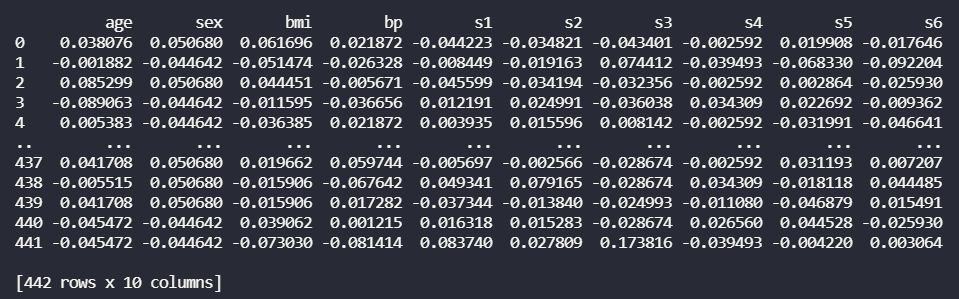In the above output, you can see the total number of rows is 442, but it displays only TEN rows. This is due to by default setting in the pandas library being TEN rows only (default number of rows may change depending on systems). Now we will see how to display all rows from the data frame using pandas.

## Method 1: Using to_string()

This method is the simplest method to display all rows from a data frame but it is not advisable for very huge datasets (in order of millions) as it converts the entire data frame into a single string. Although this works well for datasets with sizes in the order of thousands.

Syntax :

`DataFrame.to_string()`

## Python3

 `# Display all rows from data frame using pandas``# importing numpy library``import` `pandas as pd` `# importing iris dataset from sklearn``from` `sklearn.datasets ``import` `load_iris` `# Loading iris dataset``data ``=` `load_iris()` `# storing as data frame``dataframe ``=` `pd.DataFrame(data.data,columns ``=` `data.feature_names)` `# Convert entire data frame as string and print``print``(dataframe.to_string())`

Output: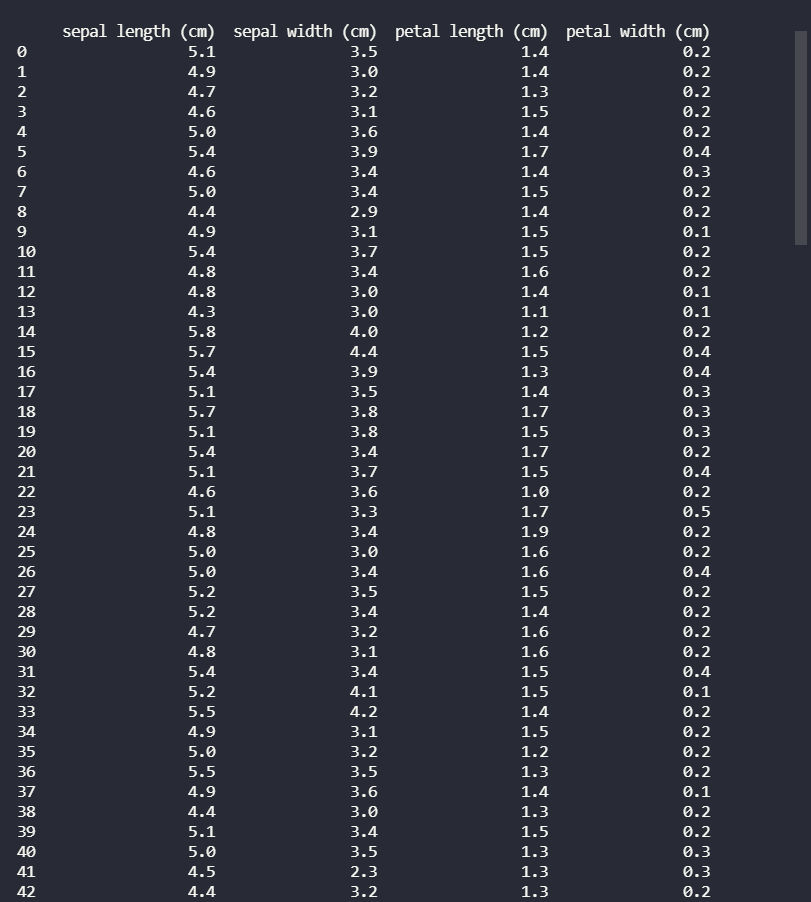Here, In the output, you can see it display all rows from the data frame. But this method is not advisable for large data frame as this convert whole data frame into a string so there might be any memory error.

## Method 2: Using set_option()

Pandas provide an operating system to customize the behavior and display. This method allows us to configure the display to show a complete data frame instead of a truncated one. A function set_option() is provided by pandas to display all rows of the data frame. display.max_rows represents the maximum number of rows that pandas will display while displaying a data frame.

The default value of max_rows is 10. If set to ‘None‘ then it means all rows of the data frame. Set value of display.max_rows to None and pass it to set_option and this will display all rows from the data frame.

Syntax :

`pandas.set_option('display.max_rows', None)`

## Python3

 `# Display all rows from data frame using pandas``# importing numpy library``import` `pandas as pd` `# importing iris dataset from sklearn``from` `sklearn.datasets ``import` `load_iris` `# Loading iris dataset``data ``=` `load_iris()` `# Default value of display.max_rows is 10 so at max``# 10 rows will be printed. Set it None to display``# all rows in the dataframe``pd.set_option(``'display.max_rows'``, ``None``)` `# storing the dataset as data frame``dataframe ``=` `pd.DataFrame(data.data,columns ``=` `data.feature_names)` `# printing data frame``print``(dataframe)`

Output: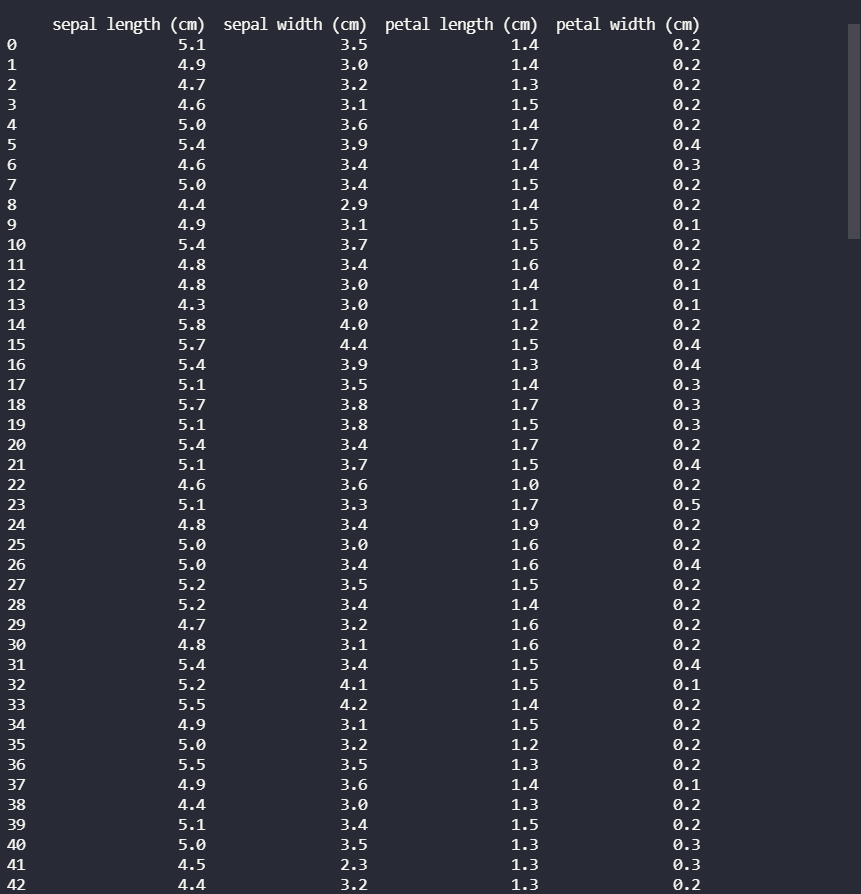## Method 3: Using to_markdown()

This method converts the data frame to a string object and adds styling and formatting to the data frame. This is the same as to_string() method but with styling and formatting added. to_markdown() will display all rows from the data frame with style and format.

Syntax :

`DataFrame.to_markdown()`

## Python3

 `# Display all rows from data frame using pandas``# importing numpy library``import` `pandas as pd` `# importing iris dataset from sklearn``from` `sklearn.datasets ``import` `load_iris` `# Loading iris dataset``data ``=` `load_iris()` `# storing the dataset as data frame``dataframe ``=` `pd.DataFrame(data.data, columns``=``data.feature_names)` `# Convert entire data frame as markdown and print``print``(dataframe.to_markdown())`

Output: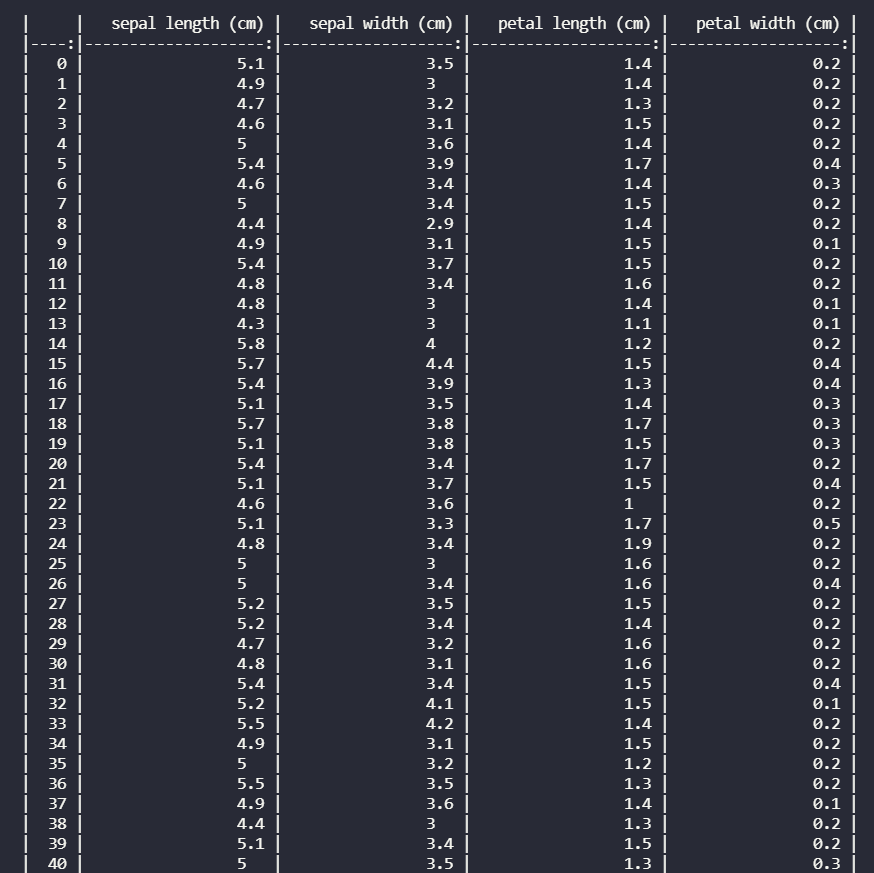## Method 4: Using option_context()

This method is identical to set_option() method. Both methods are the same only difference is set_option() change the settings permanently and option_context() former do it only within its scope. This method also uses display.max_rows as parameters and we make it equal to None to display all rows of the data frame. So, after setting the value of the display.max_rows to None and passing it to option_context we will be able to see all rows from the data frame. Its syntax is the same as set_option() method.

Syntax :

```with pandas.option_context('display.max_rows', None,):
print(dataframe)```

## Python3

 `# Display all rows from data frame using pandas``# importing numpy library``import` `pandas as pd` `# importing iris dataset from sklearn``from` `sklearn.datasets ``import` `load_iris` `# Loading iris dataset``data ``=` `load_iris()` `# storing the dataset as data frame``dataframe ``=` `pd.DataFrame(data.data, columns``=``data.feature_names)` `# The scope of these changes``# are local with systems to with statement.``with pd.option_context(``'display.max_rows'``, ``None``,):``    ``print``(dataframe)`

### Output: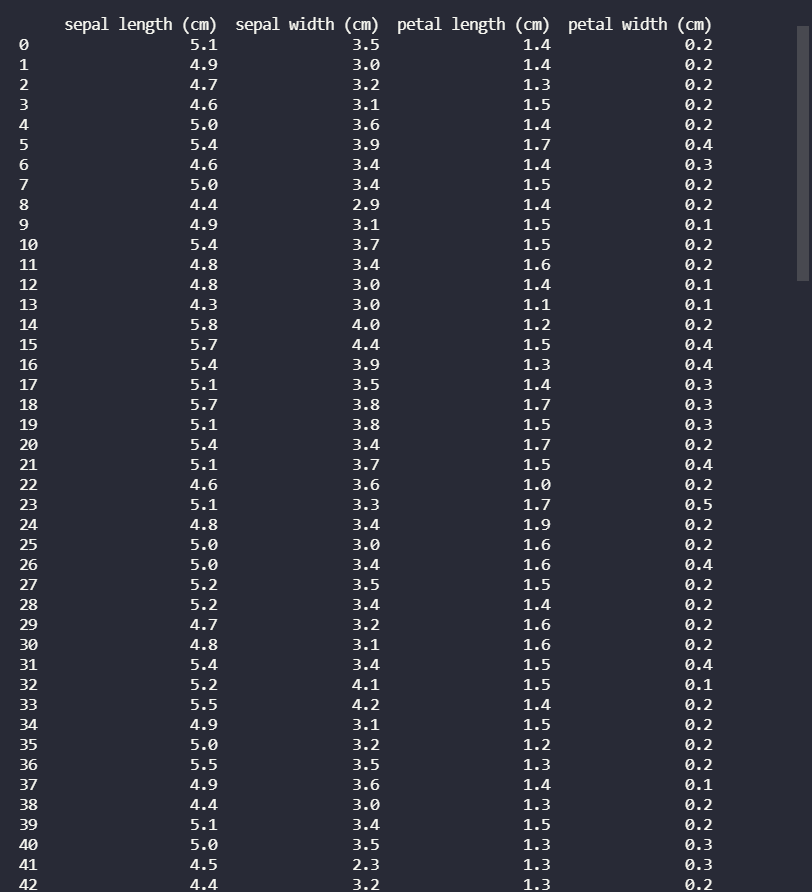My Personal Notes arrow_drop_up